Subsections

# Numerical Integration. Finite Difference methods

If we have a system of N particles, then equations (1) represent a system of 3N second-order differential equations. It can be reduced to the system of 6N first-order differential equations by introducing additional variable for the first derivative of coordinate. This leads to (2).

Both of these systems can be solved numerically using a finite difference technique. The general idea of the finite difference method is the following: given the positions, velocities, and other possible dynamic information at the time t, we attempt to obtain the position, velocities, etc. at a later time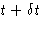with a sufficient degree of accuracy. Trajectory (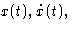etc.) is obtained by the step-by-step evaluation of these quantities. The choice of time step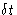depends upon the numerical integration method used and on the required accuracy. There exist many numerical methods to solve the ordinary differential equations. Unfortunately, not all of them satisfy the specific requirements of the molecular dynamics simulations:

• Large number of degrees of freedom requires the method to be easy on memory.
• The method should permit a long time step• It should satisfy the known conservation laws for energy and momentum and be time-reversible.
• Expensive force calculations practically exclude high order and predictor-corrector methods.
• The methods should be simple in form and easy to program.

## Verlet Algorithm

This algorithm, which exists in various versions, is the most popular due to its simplicity and efficiency.

Let's expand the coordinates at timesand at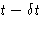: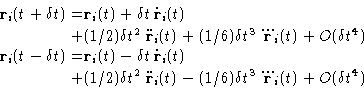(3)
By subtracting and adding them, we obtain the main equations of the Verlet algorithm: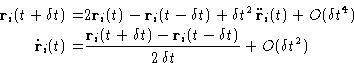(4)

Its main strength is that it allows to calculate coordinates with the accuracy of, storing only three arrays: two coordinates and accelerations, and calculating forces only once per time step. This scheme is symmetric with respect to time (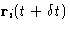and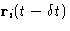enter the equations (4) symmetrically), and hence the algorithm is time reversible. Velocities are not present explicitly in this version. Although they can be calculated, but with a second order accuracy. This is inconvenient, as this introduce some additional error'' in the energy or some other properties that depend on it. Another potential drawback is that because the small, of the order of, number is added to the difference in the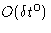values, the rounding errors may be needlessly introduced. Better estimate for the velocity can be obtained by the following modification, also known as the velocity Verlet'' algorithm, which stores the positions, velocities and accelerations at the same time t: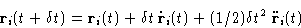(5)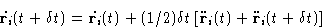(6)
Instead of storing new and old accelerations, the velocity advancement is split in two steps to save memory:

1.
Before the forces (accelerations) are updated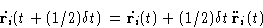(7)
and
2.
after the coordinates are updated and new forces (accelerations)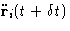are available: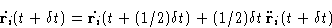(8)

## Velocity Verlet MD algorithm

1.
prepare the initial configuration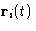and velocities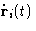2.
calculate energy and forces (accelerations)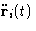, for this configuration
3.
calculate new positionsaccording to (5)
4.
calculate potential energy and forces (accelerations) at the next step